JMP uses the multivariate radial strata method for each factor that uses the Normal Weighted distribution. This seems to work better than a number of Importance Sampling methods, as a multivariate Normal Integrator accurate in the extreme tails.
First, define strata and calculate corresponding probabilities and weights. For d random factors, the strata are radial intervals as follows.
 Strata Number Inside Distance Outside Distance 0 0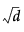1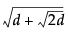2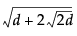i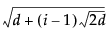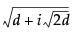NStrata – 1 previous1 Select a strata as mod(i – 1, NStrata) for run i.
 2 Determine a random n-dimensional direction by scaling multivariate Normal (0,1) deviates to unit norm.
 3 Determine a random distance using a chi-square quantile appropriate for the strata of a random uniform argument.
 4 Scale the variates so that the norm is the random distance.
 5 Scale and re-center the variates individually to be as specified for each factor.

Help created on 9/19/2017